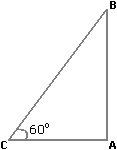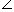# Aptitude - Height and Distance - Discussion

Discussion Forum : Height and Distance - General Questions (Q.No. 3)
3.
The angle of elevation of a ladder leaning against a wall is 60° and the foot of the ladder is 4.6 m away from the wall. The length of the ladder is:
2.3 m
4.6 m
7.8 m
9.2 m
Explanation:

Let AB be the wall and BC be the ladder.Then,ACB = 60° and AC = 4.6 m.

 AC = cos 60° = 1 BC 2BC = 2 x AC = (2 x 4.6) m = 9.2 m.

Discussion:
43 comments Page 1 of 5.

Fardeen khan said:   5 months ago
@All.

Here, actually, we want to find the (hypotenus) length of the ladder therefore we use "cos" = B/H (length's ledger).
(1)

Eben Thomas said:   9 months ago
sin=o/h , cos=a/h, tan=o/a,

Here,
o- Opposite side;
h-Hypotnuse;

Ujjawal said:   9 months ago
Here, why we have used COS?

Because we have to find the length of the ladder and the length of ladder is the hypotenuse, perpendicular is wall nd base is foot from the ladder so as we know cos equals base upon hypotenuse. So, to get results we will use this as the data given to us fits for cos.
(2)

Lorddidah said:   1 year ago
Simply remember the code SOHCAHTOA.

SOH - Sine = Opposite/Hypotenuse.
(1)

Deepali said:   2 years ago
why we take cos here? Explain please.

Yeshi Samdup said:   2 years ago
Cos60= 4.6m/hyp
0.5= 4.6m/hyp
Hyp= 4.6m/.
Therefore Hyp or length of ladder is 9.2m.
(1)

GAGAN said:   2 years ago
@Anil Kumar.

But they have asked BC not AB. Why are you finding AB?

Anil kumar behera said:   2 years ago
We all know that tan 60=AB/AC.
then, √(3)=AB/4.6,
then,4.6*√(3)=AB,
AB = 1.732 * 4.6.
AB = 7.967.
AB = 8.

Zan De19 said:   3 years ago
@All.

Take, Sin or Cos or Tan;

Sin = opposite/hypothesis

Given are as following;

The angle is 60 degree.

The foot of leader is 4.6 m away from the wall (CA) this means 4.6m is adjacent,
A leader is leaning on the wall (CB) this will be hypothesis while determining (adjacent opposite or hypothesis but in real sense, it's the length of the leader!
Now, we can make out that it's Cos and not tan or sin because adjacent CA is given (4.6m) while hypothesis CB is x or unknown.

So,
Cos60 = CA/CB.
CB(x) = 4.6/cos60.
x = 9.2.

Thank You.

Jayanth said:   3 years ago